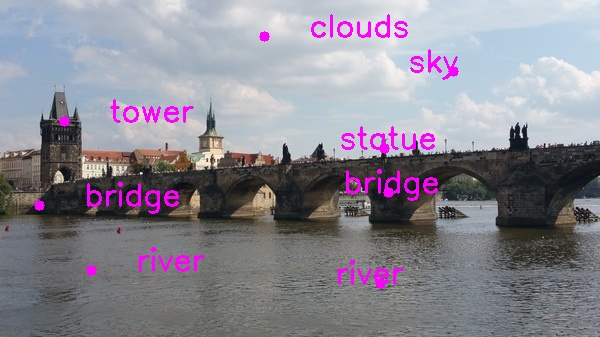# How to Annotate Images Using OpenCVIn this project, we will learn how to annotate images using OpenCV — a popular and powerful open source library for image processing and computer vision.

OpenCV is a cross-platform library with wrappers for Python, Ruby, C#, JavaScript, and other languages designed for real-time image processing. OpenCV has methods for image I/O, filtering, motion tracking, segmentation, 3D reconstruction, as well as machine learning techniques such as boosting, support vector machines, and deep learning.

# Requirements

• Design a software application using Python and OpenCV that allows users to click in an image, annotate a number of points within an image, and export the annotated points into a CSV file.
• Code must be implemented in Python and using OpenCV
• The input image and output CSV files will be provided as parameters.
• Example: python annotate_images.py cat_dog.jpg cat_dog.csv

• Python 3.7

# Directions

To run the program, open up an Anaconda Prompt terminal

Go to the proper directory.

Type python annotate_images.py cat_dog.jpg cat_dog.csv to run the program.

Here is the code:

```import cv2 # Import the OpenCV library
import numpy as np # Import Numpy library
import pandas as pd # Import Pandas library
import sys # Enables the passing of arguments

# Project: Annotate Images Using OpenCV
# Date created: 9/11/2019
# Python version: 3.7
# Description: This program allows users to click in an image, annotate a
#   number of points within an image, and export the annotated points into
#   a CSV file.

# Define the file name of the image
INPUT_IMAGE = sys.argv # "cat_dog.jpg"
IMAGE_NAME = INPUT_IMAGE[:INPUT_IMAGE.index(".")]
OUTPUT_IMAGE = IMAGE_NAME + "_annotated.jpg"
output_csv_file = sys.argv

# Load the image and store into a variable

# Create lists to store all x, y, and annotation values
x_vals = []
y_vals = []
annotation_vals = []

# Dictionary containing some colors
colors = {'blue': (255, 0, 0), 'green': (0, 255, 0), 'red': (0, 0, 255),
'yellow': (0, 255, 255),'magenta': (255, 0, 255),
'cyan': (255, 255, 0), 'white': (255, 255, 255), 'black': (0, 0, 0),
'gray': (125, 125, 125),
'rand': np.random.randint(0, high=256, size=(3,)).tolist(),
'dark_gray': (50, 50, 50), 'light_gray': (220, 220, 220)}

def draw_circle(event, x, y, flags, param):
"""
Draws dots on double clicking of the left mouse button
"""
# Store the height and width of the image
height = image.shape
width = image.shape

if event == cv2.EVENT_LBUTTONDBLCLK:
# Draw the dot
cv2.circle(image, (x, y), 5, colors['magenta'], -1)

# Annotate the image
txt = input("Describe this pixel using one word (e.g. dog) and press ENTER: ")

# Append values to the list
x_vals.append(x)
y_vals.append(y)
annotation_vals.append(txt)

# Print the coordinates and the annotation to the console
print("x = " + str(x) + "  y = " + str(y) + "  Annotation = " + txt + "\n")

# Set the position of the text part of the annotation
text_x_pos = None
text_y_pos = y

if x < (width/2):
text_x_pos = int(x + (width * 0.075))
else:
text_x_pos = int(x - (width * 0.075))

# Write text on the image
cv2.putText(image, txt, (text_x_pos,text_y_pos), cv2.FONT_HERSHEY_SIMPLEX, 1, colors['magenta'], 2)

cv2.imwrite(OUTPUT_IMAGE, image)

# Prompt user for another annotation
print("Double click another pixel or press 'q' to quit...\n")

print("Welcome to the Image Annotation Program!\n")
print("Double click anywhere inside the image to annotate that point...\n")

# We create a named window where the mouse callback will be established
cv2.namedWindow('Image mouse')

# We set the mouse callback function to 'draw_circle':
cv2.setMouseCallback('Image mouse', draw_circle)

while True:
# Show image 'Image mouse':
cv2.imshow('Image mouse', image)

# Continue until 'q' is pressed:
if cv2.waitKey(20) & 0xFF == ord('q'):
break

# Create a dictionary using lists
data = {'X':x_vals,'Y':y_vals,'Annotation':annotation_vals}

# Create the Pandas DataFrame
df = pd.DataFrame(data)
print()
print(df)
print()

# Export the dataframe to a csv file
df.to_csv(path_or_buf = output_csv_file, index = None, header=True)

# Destroy all generated windows:
cv2.destroyAllWindows()
```

# CSV Output

Here is the output for the csv file for the baby photo above: